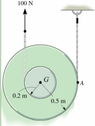# Finding Angular acceleration of the spool with the rope exerting a force on it

haven
Homework Statement:
The 7.80 kg spool has a radius of gyration of 0.320 m and rolls without the ropes slipping. The ropes have negligible mass.

There is also a force pulling the spool upward at 100N and the outer radius is 0.5 and the inner radius is 0.2-shown in picture
Let up be the positive y-direction and CCW be the positive rotational direction.
Assume 3 SF for all givens.
Relevant Equations:
Torque = I*a
Io=m*ro^2
What I did was plug in the outer radius time the force into the torque and then the mass moment of inertia is equal to m*ro^2 so then I plugged in the mass times the radius of gyration squared into I and solved for a but this is not right.

#### Attachments

•Screen Shot 2022-11-27 at 6.14.19 PM.png
37.8 KB · Views: 8

Homework Helper
Gold Member
Is there a figure that comes with this? If so, please post it using the "Attach files" link, lower left.

haven
Is there a figure that comes with this? If so, please post it using the "Attach files" link, lower left.
yes I just attached it^^